# Difference Amplifier Forms Heart of Precision Current Source

Precision current sources provide a constant current in many applications, including industrial process control, instrumentation, medical equipment, and consumer products. For example, current sources are used to provide excitation for resistance-temperature detectors (RTDs) in process-control systems; to measure unknown resistors, capacitors, and diodes in digital multimeters; and to drive 4 mA to 20 mA current loops, which are widely used to transmit information over long distances.

Precision current sources have traditionally been built using op amps, resistors, and other discrete components—with limitations due to size, accuracy, and temperature drift. Now, high-precision, low-power, low-cost integrated difference amplifiers, such as the AD8276, can be used to achieve smaller, higher performance current sources, as shown in Figure 1. The feedback buffer uses amplifiers with low offset and low bias current, such as the AD8538, AD8603, AD8605, AD8628, AD8655, AD8661, AD8663, OP177, or OP1177, depending on the required current range.Figure 1. Difference amp and op amp form precision current source.

The output current can be calculated as follows:

If Rg1 = Rg2 = Rf1 = Rf2, the equation can be reduced to:

The maximum output current is limited by the op amp input range, diff amp output range, and diff amp SENSE pin voltage range. The following three conditions must be met:within op amp input range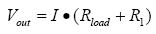within SENSE pin voltage range = 2×(–Vs) – 0.2 V to 2×(+Vs) – 3 Vwithin AD8276 output voltage range = –Vs + 0.2 V to +Vs – 0.2 V

The SENSE pin can tolerate voltages almost twice as large as the supplies, so the second limitation will be very loose. The wide 2.5-V to 36-V supply range makes AD8276 ideal for many applications. The maximum gain error of A- and B-grades is 0.05% and 0.02%, respectively, allowing current sources with up to 0.02% accuracy to be achieved.

### Configuration Variations

For cost-sensitive applications that can tolerate a little more error, the circuit can be simplified by removing the feedback buffer, as shown in Figure 2.

With

the output current is:

For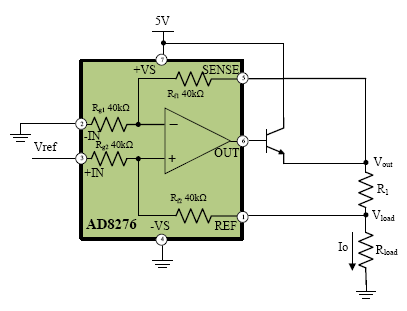Figure 2. Simplified circuit eliminates feedback amplifier.

If the required output current is less than 15 mA—the output capability of AD8276—then the boost transistor can be eliminated, as shown in Figure 3. If both low current and reduced accuracy are acceptable, the simpler, lower-cost configuration of Figure 4 can be employed.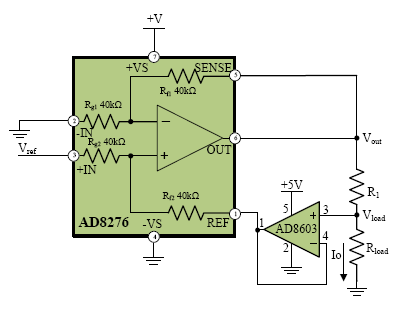Figure 3. Simplified circuit for low-current applications.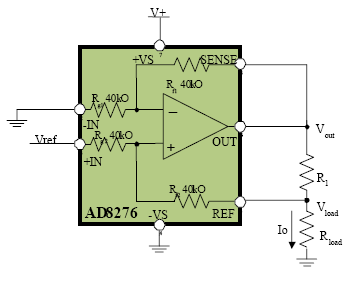Figure 4. Simplified circuit for low-cost, low-current applications.

Figure 5 shows a topology that can be used for high-current, high-accuracy applications without the limitation of op amp input range.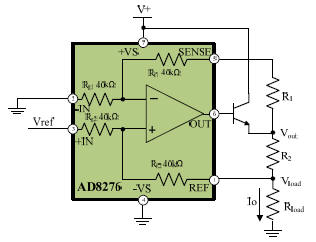Figure 5. Difference amp and matched resistors form precision current source.

The output current can be calculated as:

If ideally matched, Rg1 = Rg2 = Rf1 = Rf2 = 40 kΩ and R1 = R2, the output current is:

External resistors R1 and R2 should have ultra-high-precision and matching, or the output current will vary with the load, an error that cannot be corrected with software.

### Peripheral Components

The input voltage, VREF, can be a DAC output, voltage reference, or transducer output. If a programmable current source is needed, precision 14- or 16-bit DACs, such as the AD5640, AD5660, AD5643R, and AD5663R are recommended. For voltage references, the precision ADR42x, ADR44x are recommended for higher performance; the ADR36x is recommended for low power; the AD158x and ADR504x are recommended for low cost; and the ADR82x integrated op amp and voltage reference is recommended for small size.

The reference can connect to either the inverting or the non-inverting input of the AD8276. If using the non-inverting input, the common-mode voltage will be

and the output current will be

If using the inverting input, the common-mode voltage will be

and the output current will be

When using the inverting input, a buffer amplifier is required; the non-inverting input is thus recommended for simplicity.

### Transistor Selection

When selecting the boost transistor, make sure that VC is higher than the power supply voltage and IC is higher than the desired output current. Low cost devices, such as 2N3904, 2N4401, and 2N3391 are recommended. For lower current, the transistor is not needed.

### Experimental Bench Results and Analysis

The input voltage versus output current measured using the circuit of Figure 1 is shown in Figure 6. The AD8276 and AD8603 are powered by +5 V. The tolerance of R1 is 0.1%. The transistor is a 2N3904. The reference was swept from 0.05 V to 1.20 V with 0.01-V steps. The input range is limited by the power supply and the AD8603 input range.

The maximum error is 0.87% and the average is 0.10%. The current sense error is limited by the external resistors. Higher accuracy resistors will produce higher accuracy current sources.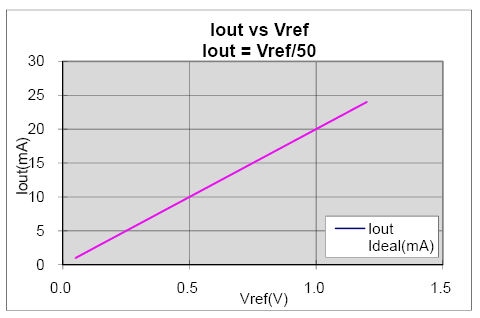Figure 6. Test result using diff amp and feedback amp

### Conclusion

The AD8276 difference amplifier—with its low offset voltage, low offset voltage drift, low gain error, and low gain drift, and integrated resistors—can be used to implement accurate, stable current sources. Its wide power supply range (2.5 V to 36 V) allows it to accommodate a wide range of loads. Its space-saving 8-lead MSOP package and its low power dissipation make it ideal for battery powered and portable systems. Implementing a precision current source with a difference amplifier can reduce PCB area, simplify layout, decrease system cost, and improve reliability.

### Appendix: Difference Amplifiers

Part 1

 Model Common Mode Range (V) Bandwidth (MHz typ) CMRR (dB) Gain Range Supply Voltage (Vmin) Supply Voltage (Vmax) AD8270 –Vs to +Vs 10 98 1.5 +5 +36 AD8271 –Vs–0.4 to +Vs+0.4 15 80 1.5 +2.5 +36 AD8273 ±40 20 86 1.5 +5 +36 AD8274 ±3 10 86 1.5 +5 +36 AD8275 –13 to +24 15 96 0.2 +3.3 +15 AD8276 2(–Vs) + 0.2 to 2(+Vs)–3 0.55 86 1 +2.5 +36

Part 2

 Model Supply Current (mA) VosTC (μV/°C) Gain TC (ppm/°C) Temperature Range Package AD8270 2.5 1.5 1 –40 to +125 LFCSP AD8271 2.6 2 2 –40 to +85 MSOP AD8273 2.5 3 2 –40 to +125 SOIC AD8274 2.6 3 0.5 –40 to +85 MSOP, SOIC AD8275 2.3 2.5 0.3 –40 to +85 MSOP AD8276 0.22 2 1 –40 to +125 MSOP, SOIC